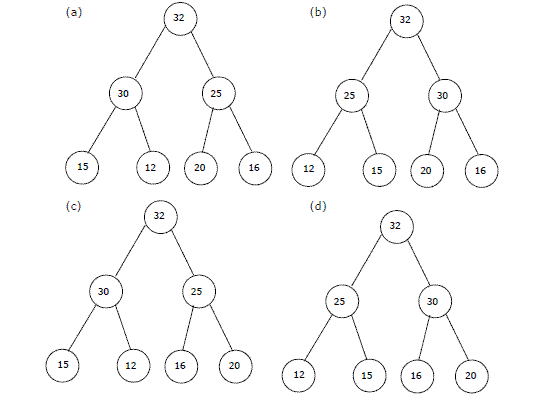# Data Structures and Algorithms | Set 5

Following questions have been asked in GATE CS exam.

1. Consider the following C function.

 `float` `f(``float` `x, ``int` `y)  ` `{  ` `  ``float` `p, s; ``int` `i;  ` `  ``for` `(s=1, p=1, i=1; i < y; i ++)  ` `  ``{  ` `    ``p*= x/i;  ` `    ``s+=p;  ` `  ``}  ` `  ``return` `s;  ` `}    `

For large values of y, the return value of the function f best approximates (GATE CS 2003)
a) x^y
b) e^x
c) ln(1 + x)
d) x^x

The function f() is implementation of Taylor’s Series to calculates e^x

```   e^x = 1 + x + x^2/2! + x^3/3! + ---
```

More is the value of y more precise value of e^x will be returned by f()

2. In the worst case, the number of comparisons needed to search a singly linked list of length n for a given element is (GATE CS 2002)
a) log 2 n
b) n/2
c) log 2 n – 1
d) n

In the worst case, the element to be searched has to be compared with all elements of linked list.

3. The elements 32, 15, 20, 30, 12, 25, 16 are inserted one by one in the given order into a Max Heap. The resultant Max Heap is.4. Consider the following three claims
I (n + k)^m = Θ(n^m), where k and m are constants
II 2^(n + 1) = 0(2^n)
III 2^(2n + 1) = 0(2^n)
Which of these claims are correct? (GATE CS 2003)

(a) I and II
(b) I and III
(c) II and III
(d) I, II and III

```(I)  (n+m)^k = n^k + c1*n^(k-1) + ... k^m = Θ(n^k)
(II)  2^(n+1) = 2*2^n = O(2^n)
```

5. A single array A[1..MAXSIZE] is used to implement two stacks. The two stacks grow from opposite ends of the array. Variables top1 and top2 (topl< top 2) point to the location of the topmost element in each of the stacks. If the space is to be used efficiently, the condition for “stack full” is (GATE CS 2004)
a) (top1 = MAXSIZE/2) and (top2 = MAXSIZE/2+1)
b) top1 + top2 = MAXSIZE
c) (top1= MAXSIZE/2) or (top2 = MAXSIZE)
d) top1= top2 -1

If we are to use space efficiently then size of the any stack can be more than MAXSIZE/2.
Both stacks will grow from both ends and if any of the stack top reaches near to the other top then stacks are full. So the condition will be top1 = top2 -1 (given that top1 < top2)